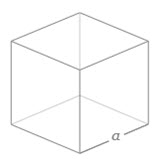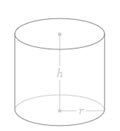Formula: A formula is nothing but a relation between two or more variables.

Subject of Formula: The subject of a formula is a variable which is expressed in terms of other variables.

Some commonly used formulas are:

 Perimeter of a square of side (a):$P=4a$$P=4a$ Area of a square of side (a):$A = a^2$$A = a^2$Volume of a cube of side (a):$A = a^3$$A = a^3$Perimeter rectangle of width ( w ) and length (l):$P = 2w + 2l$$P = 2w + 2l$ Area of a rectangle of width ( w ) and length (l):$A=w\times l$$A=w\times l$Volume of a cuboid of width (a), length (b) & height (h):$A = a\times b\times h$$A = a\times b\times h$Circumference of a circle of radius (r):$C = 2 \pi r$$C = 2 \pi r$ Area of a circle of radius (r):$A = \pi r^2$$A = \pi r^2$Surface area of a sphere of radius (r):$A = 4 \pi r^2$$A = 4 \pi r^2$ Volume of a sphere of radius (r):$\displaystyle V = \frac{4}{3}\pi r^3$$\displaystyle V = \frac{4}{3}\pi r^3$Surface area of a cone of radius (r) and height (h):$A=\pi r (r+\sqrt{h^2+r^2})$$A=\pi r (r+\sqrt{h^2+r^2})$ Volume of a cone of radius (r) and height (h):$\displaystyle V = \frac{1}{3} \pi r^2 h$$\displaystyle V = \frac{1}{3} \pi r^2 h$Surface area of a cylinder of radius (r) and height (h):$A=2 \pi rh+2 \pi r^2$$A=2 \pi rh+2 \pi r^2$ Volume of a cylinder of radius (r) and height (h):$V=\pi r^2 h$$V=\pi r^2 h$But then the formula could be any relation between variables. For example, the newton’s laws of motion as stated below$v=u+at$$2as=v^2-u^2$$S = ut+\frac{1}{2} at^2$

or something as simple like$x=2y \ or\ x=a+b$